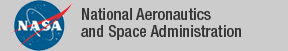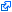### Bernstein

A formalization in PVS of an efficient representation of multivariate Bernstein polynomials has been developed. The formalization includes formally verified algorithms for finding lower and upper bounds of the minimum and maximum values of a polynomial. The algorithms are used in the definition of automated proof strategies for solving polynomial global optimization problems such as:
• Formally checking universally quantified multivariate polynomial inequalities on closed ranges.
• Finding witnesses that formally prove existentially quantified multivariate polynomial inequalities on open and closed ranges.
• Computing boxes (hyper-rectangles) that correctly under and over approximate regions defined as conjunction or disjunctions of multivariate polynomial inequalities.

• The Bernstein library is available as part of the NASA PVS Libraries.
• Grizzly is a prototype client-server tool for solving global optimization problems.

### Examples

The following examples are automatically proved in PVS using the strategy `(bernstein)`, which is included in the Bernstein library.
```  FORALL (x,y:real):
-0.5 <= x AND x <= 1 AND -2 <= y AND y <= 1 IMPLIES
4*x^2 - (21/10)*x^4 + (1/3)*x^6 + (x-3)*y - 4*y^2 + 4*y^4 > -3.4
```
```  EXISTS (x,y:real):
-0.5 <= x AND x <= 1 AND -2 <= y AND y <= 1 AND
4*x^2 - (21/10)*x^4 + (1/3)*x^6 + (x-3)*y - 4*y^2 + 4*y^4 < -3.39
```
More challenging `problems` are also included in the distribution.

### Publications

The tagidentifies links that are outside the NASA domain